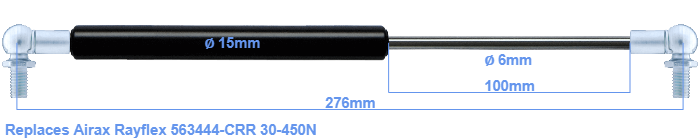# Replacement for Airax Rayflex 563444-CRR 30-450N

\$31.55

Replacement gas spring for the Airax Rayflex 563444-CRR 30-450 Newton. This fits a (possibly already present) ball with a diameter of 10mm. A corresponding ball is included. Brand: HAHN, a Stabilus company.
 Force Choose an option30 Newton40 Newton50 Newton60 Newton80 Newton100 Newton120 Newton140 Newton150 Newton160 Newton180 Newton200 Newton220 Newton240 Newton250 Newton260 Newton280 Newton300 Newton320 Newton340 Newton350 Newton360 Newton380 Newton400 Newton420 Newton440 Newton450 NewtonClear
This gas spring is also known as 5000563444-CRR, 563444-CRR.00.The diameter of the cylinder of this gas spring is 15 mm. The cross section of the rod is 6 mm. The stroke of the gas spring is 100 millimeter. The total length of this replacement gas spring is 276 mm. Attention: this is the length between the centers of the mounting parts. The distance from thread to thread (without mounting parts) is 232 mm. This gas spring (563444-CRR) has a force of 30-450 Newton. This is not an original gas spring from Airax Rayflex, but a so called replacement gas spring. This a gas spring with the brand HAHN. Nevertheless, the dimensions and force are equal.
Category: